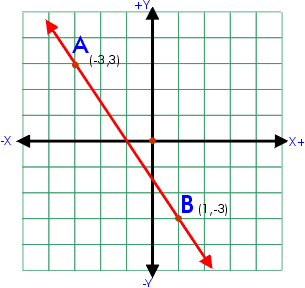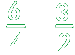The slope of a line is the ratio of its rise to its run. The rise is the vertical change between two points on a line. The difference between the y-coordinates creates the rise. In this example, the difference between the y-coordinates of points A and B is 3 - (-3) = 6. The run is the horizontal difference between two points or the difference between the x-coordinates. In this example the difference is -3 - 1 = -4.In this example, the slope of the line is.